CHAPTER 1. Mathematics Division of Whole Numbers Division is the process of finding how many times one number (called the divisor) is contained in another number (called the dividend). The result is the quotient, and any amount left over is called the remainder.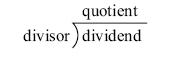Example: 218 landing gear bolts need to be divided between 7 aircraft. How many bolts will each aircraft receive?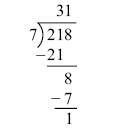The solution is 31 bolts per aircraft with a remainder of 1 bolt left over. Fractions A fraction is a number written in the form N/D where N is called the numerator and D is called the denominator. The fraction bar between the numerator and denominator shows that division is taking place.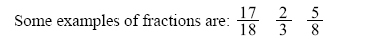The denominator of a fraction cannot be a zero. For example, the fraction 2/0 is not allowed. An improper fraction is a fraction in which the numerator is equal to or larger than the denominator. For example, 4/4 or 15/8 are examples of improper fractions. Finding the Least Common Denominator To add or subtract fractions, they must have a common denominator. In math, the least common denominator (LCD) is commonly used. One way to find the LCD is to list the multiples of each denominator and then choose the smallest one that they have in common. Example: Add 1⁄5 + 1⁄10 by finding the least common denominator. Multiples of 5 are: 5, 10, 15, 20, 25, and on. Multiples of 10 are: 10, 20, 30, 40, and on. Notice that 10, 20, and 30 are in both lists, but 10 is the smallest or least common denominator (LCD). The advantage of finding the LCD is that the final answer is more likely to be in lowest terms. A common denominator can also be found for any group of fractions by multiplying all of the denominators together. This number will not always be the LCD, but it can still be used to add or subtract fractions.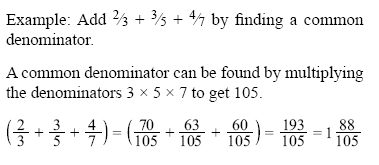Addition of Fractions In order to add fractions, the denominators must be the same number. This is referred to as having “common denominators."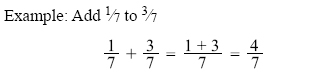If the fractions do not have the same denominator, then one or all of the denominators must be changed so that every fraction has a common denominator. Example: Find the total thickness of a panel made from 3/32-inch thick aluminum, which has a paint coating that is 1/64-inch thick. To add these fractions, determine a common denominator. The least common denominator for this example is 1, so only the first fraction must be changed since the denominator of the second fraction is already 64.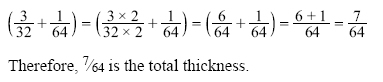Subtraction of Fractions In order to subtract fractions, they must have a common denominator.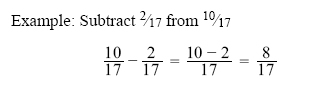If the fractions do not have the same denominator, then one or all of the denominators must be changed so that every fraction has a common denominator. Example: The tolerance for rigging the aileron droop of an airplane is 7/8 inch ± 1/5 inch. What is the minimum droop to which the aileron can be rigged? To subtract these fractions, first change both to common denominators. The common denominator in this example is 40. Change both fractions to 1/40, as shown, then subtract.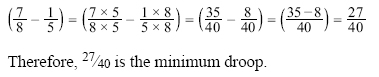©AvStop Online Magazine                                                                                                                                                      Contact Us              Return To Books